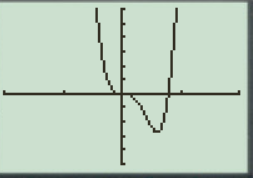# To graph : The quadratic equation y = x 4 − 4 x 3 in a graphing window.### Precalculus: Mathematics for Calcu...

6th Edition
Stewart + 5 others
Publisher: Cengage Learning
ISBN: 9780840068071### Precalculus: Mathematics for Calcu...

6th Edition
Stewart + 5 others
Publisher: Cengage Learning
ISBN: 9780840068071

#### Solutions

Chapter 1.9, Problem 19E
To determine

## To graph: The quadratic equation y=x4−4x3 in a graphing window.

Expert Solution

### Explanation of Solution

Given information:

Graph:

The graph of the quadratic equation y=x44x3 can be sketched in the cartesian plane,

Consider the quadratic equation, y=x44x3 .

Now put the values of x in the equation to find the values of y or to find the real roots of y with the help of x .

Rewrite the equation as take common:

y=x44x3y=x3(x4)

Now substitute the value y=0 in the equation y=x44x3 .

y=x3(x4)0=x3(x4)

Either x3=0 or x4=0 .

Hence x=0 and x=4 .

Now substitute the value of x=0 in equation y=x44x3 .

y=x44x3y=04403y=0

Now put the value of x=1 in the equation, y=x44x3 .

y=x44x3y=14413y=14y=3

Substitute the value x=2 in the equation y=x44x3 :

y=x44x3y=24423y=1648y=1632

Now the value of y is:

y=1648y=1632y=16

Substitute the value x=3 in the equation y=x44x3 :

y=x44x3y=34433y=81427y=81108

Now the value of y is:

y=81427y=81108y=27

Substitute the value x=1 in the equation y=x44x3 :

y=x44x3y=14413y=1+4y=5

Substitute the value x=2 in the equation y=x44x3 :

y=x44x3y=24423y=16+48y=16+32

Now the value of y is:

y=16+48y=16+32y=48

Substitute the value x=3 in the equation y=x44x3 :

y=x44x3y=34433y=81427y=81+108

Now the value of y is:

y=81427y=81+108y=189

Observed that when the value of x increases then the value of y decreases slightly.

When the value of x decreases then the value of y increases slightly.

Steps to plot the graph of the equation y=x44x3 with the help of graphing utility are as follows:

Step 1: Press MODE key.

Step 2: Use the down arrow key to reach FUNC option.

Step 3: Press ENTER key.

Step 4: Press Y= key.

Step 5: Enter the function y=x44x3 .

Step 6: Press WINDOW key. Change the settings to

Xmin=10Xmax=10Ymin=10Ymax=10

For better view of graph.

Step 8: Press GRAPH key.

The result obtained on the screen is provided below,Interpretation:

The equation of the function y=x44x3 represents a parabola.

The parabola opens downwards.

The x intercepts are the points on xaxis where the graph of the equation touches xaxis .

At x -axis y is always zero.

Recall that the graphical approach to solve the equation simultaneously.

Therefore, in the equation, y=x44x3 there is no x intercepts.

Therefore, the equation y=x44x3 is symmetric about the y axis Initially the graph of the function y=x44x3 increases when increase the value of x ,the graph is a parabola shaped graph.

### Have a homework question?

Subscribe to bartleby learn! Ask subject matter experts 30 homework questions each month. Plus, you’ll have access to millions of step-by-step textbook answers!